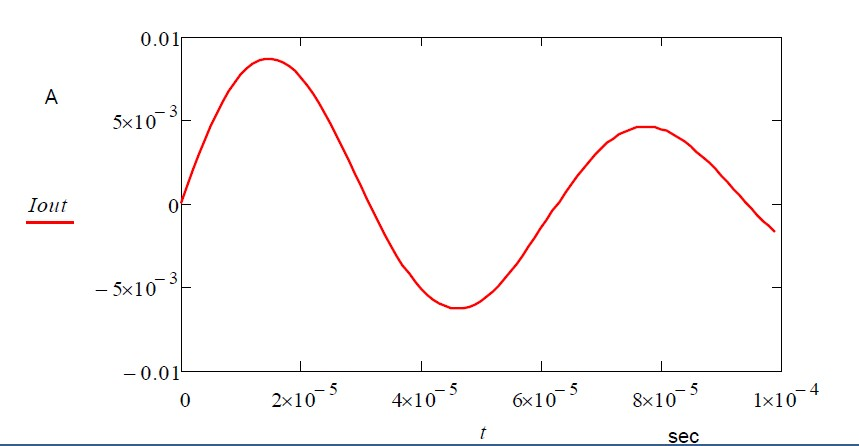## Updating Circuit Theory: Time Step Analysis

SeriesBy Ian Darney

The classical approach to time-domain analysis of EMI is to define the spectrum of the E-field, analyse the response of the system in the frequency domain and then transform the results back into the time domain. There is a simpler way; stay in the time domain.

Even so, the approach taken by academia would be to invoke the use of Laplace Transforms or Heaviside Transforms. Again, this would lead to some heavy-going mathematics.

Such complexity can be bypassed by using Time-Step Analysis. This is similar to the use of iterative techniques used to solve simultaneous equations. Simulation programs with integrated circuit emphasis (SPICE) have used this method for decades.

But there is still a fundamental problem; SPICE software is based on nodal analysis which invokes the concept of the Equipotential Ground. That is, a conductor at which all points are at zero voltage. This can be represented as a node to which all other voltages in the system are referred. Such a concept contradicts all the lessons learned from electromagnetic theory.